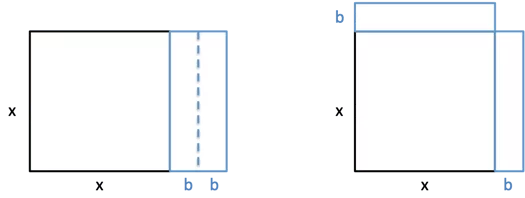Geometric and Graphical perspective on completing the square

I just read an interesting article that helps to understand completing the square, and prove the quadratic equation from a geomterical perspective.

My question is how do I understand the graphical perspectivein terms of this new geometric one• Check out James Tanton's video on youtube (3 on completing the square), and then ask him to join the conversation here. If you don't know how to contact him, I can ask after you've watched the videos. It's a great question. And if he doesn't know the answer yet, I bet he'll figure out some great connections and be grateful for the question. Apr 24 '21 at 17:20
• Really loved those videos (only saw 2), thank you! Trying to write up a full geometric proof now, maybe that will bring some insight. I Would love to hear what James has to say if that is possible. Apr 24 '21 at 18:52
• Isn't completing the square synonymous with translations that move the vertex to the origin? It takes $y=ax^2+bx+c$ and turns it into $y=a(x-h)^2+k$. Apr 24 '21 at 20:24
• @Aeryk Not sure how that is a translation, isn't that just algebraic manipulation? Vertex-form does seem interesting, please correct me if I am misunderstanding Apr 24 '21 at 21:14

Note: as in the originally referenced article, I'll take $$a=1$$ to keep it less complicated.

I think it's important to first establish two key issues about the graphical setting:

1. What does it mean to "be a square" in a graph.
2. What information are we assuming we can "read" from the graph.

For (1), in the geometric setting this notion is obvious (e.g. rectangle with all equal sides or the area of such) and algebraically it's something of the form $$(\text{stuff})^2$$. Motivated by these ideas, it seems that graphically to "be a square" should be to be parabolic with vertex on the $$x$$-axis. (Or perhaps even vertex at the origin.)

For (2), let's assume we can obtain the coordinates of the vertex, $$(h,k)$$. Or, if you prefer, the focus and the directrix that define the parabola initially, but from them we can find the vertex as the midpoint between the focus and the directrix.

Putting (1) and (2) together, we can "complete the square" by a vertical translation of $$k$$ units which results in a parabola with vertex on the $$x$$-axis.

For example, given the graph of $$y=x^2-x-2$$ we find that the vertex is at $$(1/2,-9/4)$$. So we subtract $$-9/4$$ from both sides to get $$y-\left(-\frac{9}{4}\right) = x^2-x-2-\left(-\frac{9}{4}\right)$$ $$y+\frac{9}{4} = \left(x-\frac{1}{2}\right)^2$$ Notice, of course, that what is being squared is $$x-h$$.

Looking at this I would probably agree with the criticism that it seems a little too trivial and it's not nearly as cool or demonstrative as the geometric scenario. However, I think for students it could be a good example of how physicists/mathematicians/scientist often change coordinate systems to make equations easier. What once was $$y=x^2-x-2$$ becomes $$y=x^2$$ after a vertical and horizontal shift. So we were just measuring from the "wrong" origin to begin with.

I say that the story of quadratics -- their algebra and their graphing -- is a beautiful story of the of power of symmetry. If I have a rectangle of area 36, you know little about that rectangle: it was area 36. But if I add I have a (maximally) symmetrical rectangle of area 36, then you know everything about it: it must be a 6-by-6 square. The mention of symmetry collapses uncertainty into certainty here. That's the power of the "quadrus" method (the "square" method) that allows you to solve equations of the form $$ax^2 + bx + c = q$$, aka quadratic equations. (Get the name?)

This algebraic method leads you to see that any graph of an equation $$y = ax^2 +bx + c$$ is going to be the same beautiful symmetric shape as the graph of $$y = ax^2$$. Ahh ... symmetry again! Let's use it to our advantage!

Graph: $$y = (x-2)(x-6)+7$$. Okay. I see $$x=2$$ and $$x=6$$ staring me in the face. They each give $$y = 7$$. Oh! I have two symmetrical points on a symmetrical graph. The line of symmetry must be at $$x=4$$, halfway between. At $$x=4$$, $$y=3$$. Oh! The graph is now clear.

Graph: $$y = 2x^2 + 8x +5$$ Okay. $$y =2x(x+4) + 5$$. I see $$x=0$$ and $$x=-4$$ both give $$y=5$$. Ahh. Line of symmetry is at $$x=-2$$. Put in this value and the graph of the equation becomes clear. The power of symmetry!

Yes https://www.youtube.com/channel/UCib_J32VI8rQI_LCFXn1XAA has the videos and here is a PDF of everything, in case you are interested: http://www.jamestanton.com/?p=2296.

p.s. The graph of y = x^2 is a symmetrical "U"-shaped graph. If you add something non-symmetrical to it, like 2x+1, and so graph y = x^2+2x+1, it should be a shock to see the same symmetrical U -shape graph appear! Seriously, whoa! But the algebra of completing the square shows this is really the graph of y= (x+1)^2 with x=-1 behaving like zero. In general, as people are showing, the algebra of completing the square gives, for example:

y = 2x^2 - 4x + 1

2y = 4x^2 - 8x + 2 (I am following my nose after drawing a picture of a square and wishing for easy numbers)

2y+2 = 4x^2 - 8x + 4

2y+2 = (2x-2)^2 = 4(x-1)^2 (looking at my picture)

y = 2(x-1)^2 - 1

Yep... it is just y=x^2 again, made steeper, with x=1 behaving like zero, and everything shifted down one.

This stuff is really about teaching kids the confidence to follow their wits and commonsense. (If I really needed a graph of y = 2x^2 - 4x + 1 I would just type it into Desmos ... So what is this material teaching really in the 21st century?)